Shade.ca Web DesignRandom Review3DMOL - The mathematics

 Before we start, we must remind you that the camera points in the same direction as the Z axis and that the points to draw are in front of it. We will see where the points would be projected on the surface that is used as the projection screen. The formula that we will find will be independent from the dimensions of the screen, we will use these dimensions later on while wanting to find which points are in the cameras field of view.     The camera is kind of a rectangular based pyramid where the peak of the pyramid is set at the origin (0,0,0) and the base serves as a projection screen for the 3D universe. What the user will see will be the image of this 3D world on the rectangular base. The focal distance of the camera is the distance between the origin (0,0,0) and the surface which contains the base of the pyramid.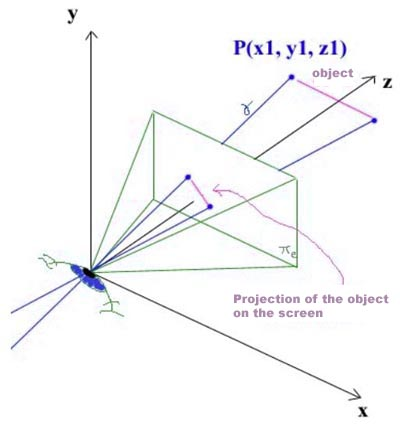(representation #2, the projection screen)     On this graphic, the green pyramid is the camera with its projection screen, the purple line is a 3D object to be projected on the 2D screen and the blue lines will help us find where our object should be on the projection screen.         The surface PI(e): Ax + By + Cz + D = 0         The 3D point we will transform to 2D: P(x1,y1,z1) Plane representing the screen     Usually, the normal to a surface does not have a specific length. However, we have assigned a length (which is equal to the focal distance) to the vector N, the normal of the plane PI(e). To simplify our equations, we will call N the length of the vector N and, at the same time, the focal distance.     N=(0,0,N) because the camera points in the same direction as the vector k.     We must define PI(e) more accurately using N and the point (0,0,N), an element of PI(e). PI(e):     0x + 0y + Nz + D =0     0x + 0y + N*N + D = 0        after inserting the point (0,0,N) in the equation     D = - N*N(formula 1, plane of the camera)     It would also be correct to say that the equation of the plane is z=N, but it is easier to see that it is a plane without this simplification. Line crossing the screen     The directing vector of the line passing through the origin (0,0,0) and the point P(x1,y1,z1) will be the vector (x1-0,y1-0,z1-0).     The line named y passes by the origin and P(x1,y1,z1):         x=x1*t + 0         y=y1*t + 0         z=z1*t + 0     The intersection of this line y with the plane PI(e) will be defined by the equation 0(x1*t) + 0(y1*t) + N(z1*t) - N*N=0     Therefore, we find that t = (N*N)/(N*z1) = N/z1 when y crosses PI(e).     By taking the previous formulas, we see that the coordinates of the point where y crosses PI(e) (location of the 2D projection) are defined by: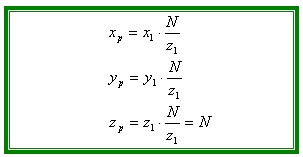(formula 2, points of crossing) Computer screen problem     It is very useful to finally have our formulas to pass from a 3D to a 2D world, but without the correct conversions to display them on the computer screen, they are useless. A computer screen is made as to have the upper left corner being the origin of the surface. The values of the x axis grow when moving to the right and the values of the y axis grow while going down the screen. We needed to find certain formulas to convert our 2D points on our virtual rectangle to the concrete points we wish to display to the computer screen. These formulas are linear transformations of the formula number 2 (see above) and have been verified with a little experimentation.         P(x1,y1,z1) is the point in 3D to be displayed on the computer screen,             P(xc,yc) is the point once transformed             Sw is the screen width,             Sh is the screen height,             N is the focal distance,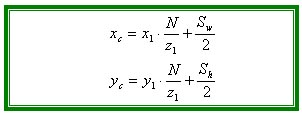(formulas 3, coordinates on the computer screen)     When it comes time to display the points on the screen, we verify if they are actually on the screen and not in the plane that contains the surface. Currently, computer monitors are usually set to a resolution of 800 pixels wide and 600 pixels high. We only need to verify that 0 < Xc < 800 and that 0 < Yc < 600. If a point does not respect these rules, the software ignores them and they are not displayed. Furthermore, if the z coordinate of the point is smaller than the focal distance, the point is not in front of the camera and will therefore not be displayed. By ignoring these points, we do not overload the computer with useless display instructions. Formulas for 3D rotations around one axis A few formulas used throughout the demonstrations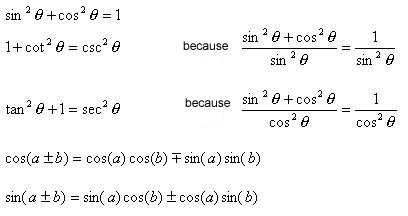Demonstration of 2D rotations     A 3D rotation is the same thing as doing three in 2D, with each being around a different axis. First, we will learn how to do a rotation around the z axis. It will then be simple to adapt the formula for the other axes. We only need to take the coordinates in Y and Z to rotate around the X axis, the X et Z coordinates to rotate around the Y axis and the X and Y coordinates to rotate around the Z axis. Because we do not need the initial angle between the point to be turned and the origin, we have more freedom while trying to find the formula.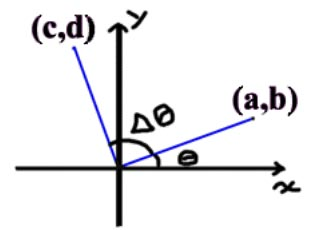(representation 3, 2D rotation around the Z axis)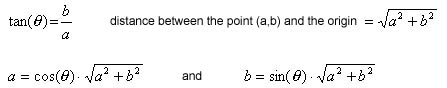To find the "c" coordinate from delta theta, a and b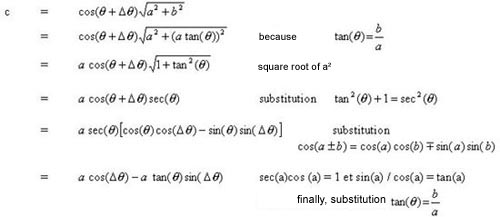(formula 4, the first coordinate after rotation)     To find the "d" coordinate from delta theta, a and b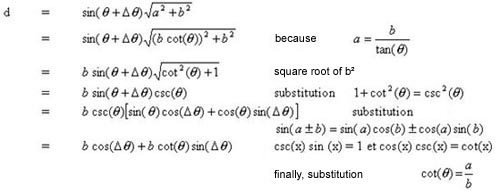(formula 5, the second coordinate after rotation) Inversed rotation     To change the direction of rotation in the formulas, we could apply a rotation of -Theta and transform the formulas (because cos and sin are respectively even and odd functions) to obtain new formulas that apply rotations in the opposite direction. Specific formulas     Once again, let us repeat the initial coordinates (a,b) will be composed of the pair of coordinates for which we will not turn around the axis. Therefore, here are the formulas: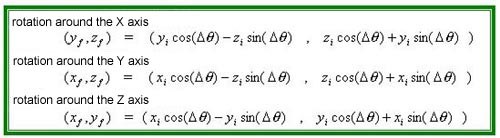(formulas #6, rotation around the axes)     These formulas could also be expressed using transformation matrices.It would therefore be possible to compose a 3x3 matrix that could apply all of the above rotations by multiplying these matrices from the left. We also notice that the determinant of these matrices is always 1. However, we currently ignore what this implies. Face sorting     While creating our objects, it was very important to define our points in a counter-clockwise order and we will now explain why. It is important to mention that we did not find this little trick on our own and that we tried a few ideas before asking Jérôme St-Louis (the author of a page we consulted to realize our project) for his help. Algorithm     To know if a face points towards us, we need to do the mixed product of the three vectors formed by three consecutive points of the face and the origin. As mentioned previously, we chose to use a z axis in the opposite direction as the one we would normally have. If the z axis would have been normal, the face would point towards us if the volume (mixed product) was positive. However, because we inversed the z axis, the face needs to be drawn if the mixed product is negative.(formula #7, hidden surface removal)(representation 4, hidden surface removal)     We use a triangular based pyramid to explain the theory behind this formula. The vectors v1, v2 and v3 touch the corners of the base of the pyramid. The dot product of v1^v2 gives us a vector pointing towards the right in our representation. If we can see the base of the pyramid, v3 must be beneath the surface that includes v1 and v2 because these vectors are defined in a counter-clockwise manner. Therefore, the dot product between v1^v2 and v3 will be negative. We call a mixed product a cross product followed by a dot product. A mixed product is also the determinant of a 3x3 matrix formed by the three vectors in question. Hence, the face is hidden if the mixed product if positive. A note from the authors - Introduction - The applicationThe mathematics behind the project. - Discussion

 Jason  ( 2001-05-24 )